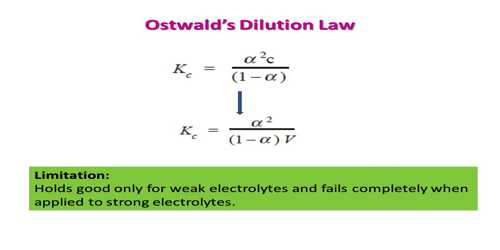Chemistry

# Dissociation of weak acids and weak bases: Ostwald’s Dilution LawDissociation of weak acids and weak bases: Ostwald’s Dilution Law

Weak electrolytes are incompletely dissociated into ions. Ostwald’s dilution law is the application of the law of mass action to weak electrolytes in solution. Consider the equilibrium involving the dissociation of the binary electrolyte MA:

MA(aq) ↔ M+ (aq) + A (aq)

The equilibrium constant, KC, is given by,

KC = [M+][A] / [MA] … … (1)

If the concentration of the electrolyte is C mol L-1 and α is the degree of dissociation,

[M+] = [A] = αc

and, [MA] = (1 – α) c

These on substitution in equation (1) give,

KC = α2c / (1 – α) … …. …. (2)

At a constant temperature, KC has a constant value. Hence when the concentration ‘c’ is changed ‘a’ must change in order to keep the value of KC constant. Equation (2) is called the ‘Ostwald Dilution Law’. The higher the value of KC, the stronger the electrolyte. This equation represents the variation of the degree of dissociation with concentration.